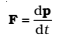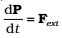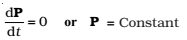The linear momentum of a particle is defined as

p = m v

Let us also recall that Newton’s second law written in symbolic form for a single particle isFor the system of n particles, the linear momentum of the system is defined to be the vector sum of all individual particles of the system,

P = p1 + p2 +…..+ pn

P = m1v1 + m2v2 +…..+ mnvn

Comparing this with eq(4)

P = M V

Thus, the total momentum of a system of particles is equal to the product of the total mass of the system and the velocity of its centre of mass.

Differentiating this eq P = M V , with respect to time,………… (7)

Comparing Eq.(7) and Eq.(6),……………(8)

This is the statement of Newton’s second law extended to a system of particles.

Suppose now, that the sum of external forces acting on a system of particles is zero. Then from Eq.(8)